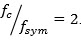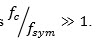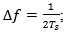## Açıklama

1. Consider a channel responseFirst obtain the analog response by spline interpolating between the discrete sample values. Then plot the resulting

h(t) in time domain by assuming a reasonable value of the Samples_per_Bit = SpB,for example 100 or more samples per symbol (bit) interval in order to get a nice plot. . Notice that your impulse response for 7 bit intervals.

Secondly, consider the 7-bit input message b-3b-2b-1b0b1b2b3=1101100  The bold pixel is the current bit (k=0). Now encode this message as a sequence of rectangular pulses. Make sure that the logic 0 is encoded as -1 and logic 1 as +1 (bipolar encoding). Now plot the overlaid output waveforms (do not sum them) over the 7-bit intervals. Try using a different color for the response to each bit pulse, say, blue, red, dark brown and black , respectively, for instances

k=±3,k=±2,k=±1,k=0 Your plot length should gain be lasting for 7 bit intervals.

2. In this part, you are supposed to draw the eye diagram of the receiver (Read Section  7.6 from Lathi 4th edition). To do that, you can follow two methods.
Method A:

First generate all 128 possible 7-bit bit combinations. Then superpose all possible received signals under ISI during the k=0 bit interval on a single plot. In other words you should add for each received waveform the interferences due to the precursors and postcursors of b0.   Any one bit sequence pattern from 0000000 to 1111111 will generate a different ISI pattern. Superimpose on your plot all these 27=128 waveform combinations. In effect you are simulating what you would be seeing on an oscilloscope if you were to trigger the horizontal line at the pulsing rate. This is the eye diagram of the receiver.

Method B:

Consider a long sequence of randomly generated bits, e.g., N = 256 long. You should create a vector that is NxSpB sample long, e.g., 256 x 100 = 25600. For

• Set all vector elements to zero

• Bit #1: Add the impulse response samples to the first 7xSpB = 7×100 = 700 components of the vector;
• Bit #2: Add the impulse response samples to the vector components indexed 101 to 800 or in general, from (SpB+1) to (7+1)xSpB

• And so on.

• Now, in order to create the eye pattern, create one plot with SpB samples (e.g., 100). You chop the NxSpB (e.g., 25600) long sequence into segments of SpB (e.g., 100) samples, and plot each one on the same interval, that is, superpose all the plots. .

• This should be your eye diagram, an indicator of the health of the channel for digital communication.

II. SIGNAL CONSTELLATIONS
In this part you are supposed to plot symbol waveforms for different signal constellations. Consider the base waveform b(t)= cos(wt) takeIn other words each  symbol should contain  two periods of b(t) as we used to draw on the board. In practice , of course ,one hasTake the symbol period as T sym=1

In MATLAB, you could take the Samples_per_Symbol = SpS as =100, that is, each symbol will be represented at least by 100 samples. . Draw the waveforms in the signal constellations for:

a. Antipodal BPSK signals

c. 4-FSK signals where the separation between frequencies is the minimum possible, that is,d. QPSK signals;

e. 16-QAM signals (plot the 16 signals by 4×4 subplotting);

In each case the transmitter power P is fixed, hence energy per symbol is